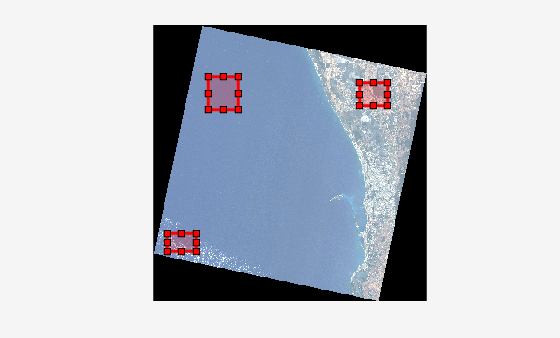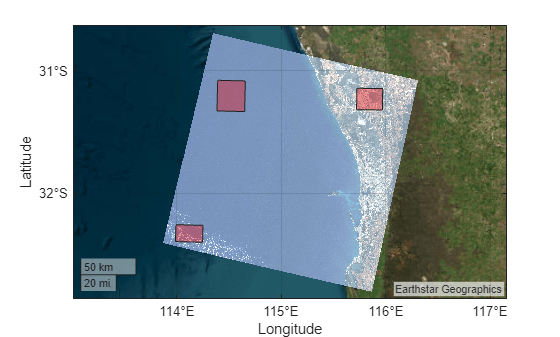# Manually Label ROIs in Multispectral Image

This example shows how to manually select regions of interest (ROIs) from a multispectral image and save them in a shapefile using the Mapping Toolbox™.

This example requires the Image Processing Toolbox™ Hyperspectral Imaging Library. You can install the Image Processing Toolbox Hyperspectral Imaging Library from Add-On Explorer. For more information about installing add-ons, see Get and Manage Add-Ons. The Image Processing Toolbox Hyperspectral Imaging Library requires desktop MATLAB®, as MATLAB® Online™ and MATLAB® Mobile™ do not support the library.

Many supervised learning applications require labeled training data. This example shows how to manually label multispectral or hyperspectral images by selecting ROIs and saving them in a shapefile. You can use the shapefile to train deep learning networks.

In this example, you perform these steps:

1. Read a multispectral image and select multiple ROIs.

2. Convert the ROIs into geographic coordinates.

3. Save the geographic coordinates of the ROIs in a shapefile.

4. Read the shapefile and visualize the ROIs in a geographic axes.

Landsat 8 is an Earth observation satellite that carries the Operational Land Imager (OLI) and Thermal Infrared Sensor (TIRS) instruments.

The Landsat 8 data set has 8 spectral bands with wavelengths that range from 440 nm to 2200 nm. The data is 7721-by-7651 pixels in dimension with a spatial resolution of 30 meters.

Download the data set and unzip the file by using the `downloadLandsat8Dataset` helper function. The helper function is attached to this example as a supporting file.

```zipfile = "LC08_L1TP_113082_20211206_20211206_01_RT.zip"; landsat8Data_url = "https://ssd.mathworks.com/supportfiles/image/data/" + zipfile; downloadLandsat8Dataset(landsat8Data_url,pwd)```
```Downloading the Landsat 8 OLI dataset. This can take several minutes to download... Done. ```

Read the Landsat 8 multispectral data into the workspace as a `hypercube` object.

`hCube = hypercube("LC08_L1TP_113082_20211206_20211206_01_RT_MTL.txt");`

Estimate an RGB image from the data cube by using the `colorize` function. Apply contrast stretching to enhance the contrast of the output RGB image.

`rgbImg = colorize(hCube,Method="rgb",ContrastStretching=true);`

Adjust the intensity values of the image for better visualization using the `imadjustn` function.

`rgbImg = imadjustn(rgbImg);`

Read the spatial referencing information for the Landsat 8 data from the corresponding GeoTIFF image.

`info = georasterinfo("LC08_L1TP_113082_20211206_20211206_01_RT_B1.TIF");`

Calculate the data region using the corner coordinates of the GeoTIFF image.

```R = info.RasterReference; xlimits = R.XWorldLimits; ylimits = R.YWorldLimits; dataRegion = mappolyshape(xlimits([1 1 2 2 1]),ylimits([1 2 2 1 1])); dataRegion.ProjectedCRS = R.ProjectedCRS;```

### Select ROIs and Save in Shapefile

Specify the number of ROIs to select. For this example, select three ROIs.

`numOfAreas = 3;`

Visualize the estimated RGB image. Use the `pickPolyshape` helper function, defined at the end of this example, to select rectangular ROIs and store the x- and y-coordinates of the ROIs in the cell arrays `polyX` and `polyY`, respectively.

```figure imshow(rgbImg) polyX = cell(numOfAreas,1); polyY = cell(numOfAreas,1); for ch = 1:numOfAreas [x,y] = pickPolyshape(R); polyX{ch} = x; polyY{ch} = y; end```Create ROI shapes from the ROI coordinates by using the `mappolyshape` (Mapping Toolbox) function.

```shape = mappolyshape(polyX,polyY); shape.ProjectedCRS = R.ProjectedCRS;```

Create a geospatial table from the ROI shapes.

`gt = table(shape,VariableNames="Shape");`

Write the ROI shapes to the shapefile format. You can use this shapefile as labeled data.

`shapewrite(gt,"Landsat8ROIs.shp")`

### Read Shapefile and Visualize ROIs in Geographic Axes

Read the shapefile as a geospatial table.

```S = readgeotable("Landsat8ROIs.shp"); S.Shape.ProjectedCRS = R.ProjectedCRS;```

Visualize the ROIs in a geographic axes along with the data region of the Landsat 8 multispectral image.

```figure geoplot(dataRegion) hold on geobasemap satellite geoplot(S)```### Supporting Functions

The `pickPolyshape` helper function performs these tasks:

1. Creates a customizable rectangular ROI.

2. Calculates the x- and y-coordinates of the corners of the ROI.

3. Transforms the intrinsic coordinates of the ROI to world coordinates.

```function [xWorld,yWorld] = pickPolyshape(R) roi = drawrectangle(Color="r"); x1 = roi.Position(1); y1 = roi.Position(2); x2 = x1 + roi.Position(3); y2 = y1 + roi.Position(4); [xWorld,yWorld] = intrinsicToWorld(R,[x2 x1 x1 x2 x2],[y1 y1 y2 y2 y1]); end```